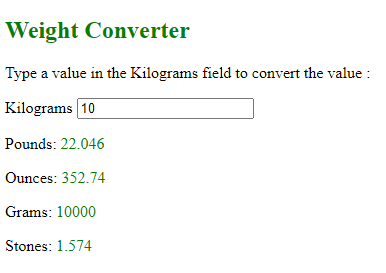Related Articles

# How to create a weight converter with HTML and JavaScript ?

• Last Updated : 27 Sep, 2021

Introduction:

What we generally call “weight” in our daily lives is theoretically referred to as mass, and it calculates the amount of matter in an object. But we can use mass and weight almost interchangeably by simply multiplying or dividing it by the Earth’s gravitational acceleration, g and this is because of gravitational force the Earth exerts on us is approximately constant. To prevent misunderstanding or unnecessary confusion, we’re talking about mass units, but we’re referring to them as weight units.

The weight converter helps you convert between kilograms, grams, pounds, ounces, and stones. All of which are measurements of weight and mass. This is very useful for beginners to train their logic in javascript.

Create a Weight Converter

First you have to create an input field to convert a value from one weight measurement to another.

## HTML

 `<``p``>``  ````  ``<``label``>Kilograms``  ````  ````  ``<``input` `id``=``"Kilograms"` `type``=``"number"` `placeholder``=``"Kilograms"``  ``oninput``=``"kiloweightConvert(this.value)"``     ``onchange``=``"kiloweightConvert(this.value)"``>```

The <input> tag use as an input field where the users can enter their data and placeholder is attribute that specifies a short hint that describes the expected value of an <input> element to be enter by user.

The oninput is a event occurs when an element gets user input i.e. when the value of an <input> or <textarea> element is changed. In above code this attribute contains value “kiloweightConvert(this.value)” and it works when oninput event triggered.

The onchange event is occur when the value of an element has been changed.

These both events are similar but the difference is that the oninput event gets activated after the value of an element has changed, while onchange event occurs when the element loses its focus, after the content has been changed. The onchange event is also works on <select> elements.

Second, create an output field for result of the conversion.

## HTML

 `` `<``p``>Pounds: <``span` `id``=``"Pounds"``>`  `<``p``>Ounces: <``span` `id``=``"Ounces"``>`  `<``p``>Grams: <``span` `id``=``"Grams"``>`  `<``p``>Stones: <``span` `id``=``"Stones"``>`

<span> tag is a generic inline container for inline elements and content which is used to mark up a part of a text, for grouping and applying styles to inline elements.

Now, it’s time for build functionality using javascript . The following function will evaluate the value and return result.

## Javascript

 `//function that evaluate the value and returns result``function` `kiloweightConvert(value) {``  ``document.getElementById(``"Pounds"``).innerHTML=value*2.2046;``  ``document.getElementById(``"Ounces"``).innerHTML=value*35.274;``  ``document.getElementById(``"Grams"``).innerHTML=value*1000;``  ``document.getElementById(``"Stones"``).innerHTML=value*0.1574;``}`

If you use innerHTML, you can modify the content of the page without refreshing it. This will make the website more responsive and quicker to user inputs. The innerHTML property can also be used along with getElementById() in  JavaScript code to refer to an HTML element and modify its contents. The method getElementById() returns an element object that represents an element whose id property matches the specified string.

You can also try the following measurements to make a weight converter.

To get your head around the conversions between different units, feel free to use the weight conversion chart given below. This  conversion table  will be very useful in designing the weight converter according to your preferences.

Example: Given below shows how  to convert a value from Kilogram to other measurements:

## HTML

 ```<``html``>``    ``<``head``>``        ``<``title``>Weight Converter``        ````        ``<``style``>``            ``span {``                ``color: Green;``            ``}``        ````    ````    ``<``body``>``        ````        ``<``h2` `style``=``"color: Green;"``>Weight Converter``        ` `<``p``>Enter a value in the Kilograms field to convert :`  `        ` `<``p``>``            ````            ``<``label``>Kilograms``            ````            ````            ``<``input` `id``=``"Kilograms"``                   ``type``=``"number"``                   ``placeholder``=``"kilograms"``                   ``oninput``=``"kiloweightConvert(this.value)"``                   ``onchange``=``"kiloweightConvert(this.value)"` `/>``        ``` `        ````        ` `<``p``>Pounds: <``span` `id``=``"Pounds"``>` `        ` `<``p``>Ounces: <``span` `id``=``"Ounces"``>` `        ` `<``p``>Grams: <``span` `id``=``"Grams"``>` `        ` `<``p``>Stones: <``span` `id``=``"Stones"``>`  `        ``<``script``>``     ``//function that evaluates the weight and return result``            ``function kiloweightConvert(value) {``                ``document.getElementById("Pounds").innerHTML =``                  ``value * 2.2046;``                ``document.getElementById("Ounces").innerHTML =``                  ``value * 35.274;``                ``document.getElementById("Grams").innerHTML =``                  ``value * 1000;``                ``document.getElementById("Stones").innerHTML =``                  ``value * 0.1574;``            ``}``        ````    `````

Output:Hey geek! The constant emerging technologies in the world of web development always keeps the excitement for this subject through the roof. But before you tackle the big projects, we suggest you start by learning the basics. Kickstart your web development journey by learning JS concepts with our JavaScript Course. Now at it’s lowest price ever!

My Personal Notes arrow_drop_up